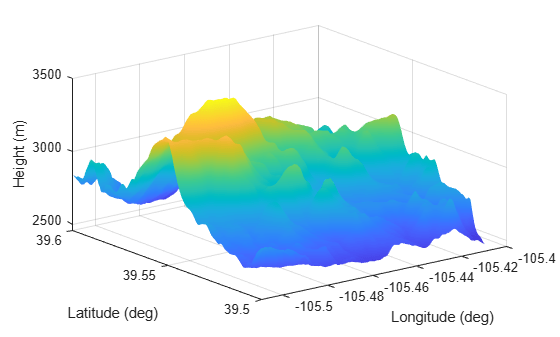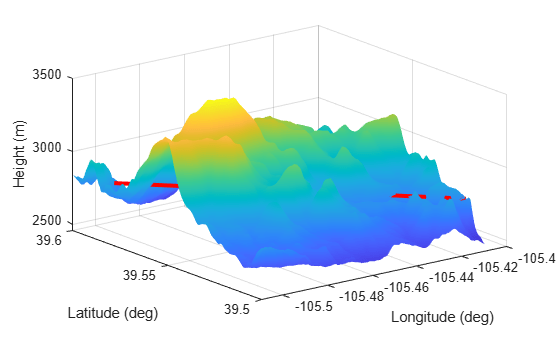# LandSurface

Land surface belonging to radar scenario

Since R2022a

## Description

`LandSurface` defines a land surface object belonging to a `radarScenario` object. You can use the `LandSurface` object to determine land heights in a scenario and surface reflectivity, test for occlusion along the line-of-sight between two points in the scenario and give surface height at a point.

## Creation

Create `LandSurface` objects using the `landSurface` object function of the `radarScenario` object.

## Properties

expand all

Radar reflectivity object of surface, specified as a `surfaceReflectivityLand` or `surfaceReflectivityCustom` System object™ for the normalized radar cross section (NRCS) of the surface. Defaults to a `surfaceReflectivityLand` object using a Barton land model and flatland land type.

Example: `surfaceReflectivityLand(Model="GIT",LandType="Soil",SurfaceHeightStandardDeviation=1)`

This property contains a grid of reflectivity type values corresponding to vertices of the surface height data. If any terrain or a spectral model is present, `ReflectivityMap` must be a matrix of the same size as the domain of that data. Otherwise it must be scalar. Each element is an index into the third dimension of the `Reflectivity` property of the `surfaceReflectivityCustom` object.

#### Dependencies

To enable this property, set the `RadarReflectivity` property to a `surfaceReflectivityCustom` object.

Data Types: `double`

Reference height of surface height data, specified as a scalar. Surface heights are relative to the reference height. For surfaces with no height data, this is the assumed height of the entire surface. Units are in meters.

Data Types: `double`

Bounding rectangle of the surface, specified as a 2-by-2 matrix of real values. The bounding rectangle is defined by two two-dimensional points in either Cartesian or geodetic scenario coordinates. When the `IsEarthCentered` property of the `radarScenario` object is specified as:

• `false` — Scenario coordinates are Cartesian. Specify the bounding rectangle [`MinX`, `MaxX`, `MinY` `MaxY`], as Cartesian coordinates in the reference frame of the scenario, where `MinX` < `MaxX`, and `MinY` < `MaxY`.

• `true` — Scenario coordinates are geodetic. Specify the bounding rectangle as [`StartLat`, `EndLat`, `StartLon` `EndLon`] where `StartLat` and `EndLat` are the minimum and maximum latitudes of the geodetic frames. `StartLat` and `EndLat` must lie in the interval [–90,90] where `StartLat` < `EndLat`.

Specify `StartLon` and `EndLon` as the minimum and maximum longitudes of the geodetic frame. `StartLon` and `EndLon` must lie in the interval [–180,180]. If `EndLon` < `StartLon`, the object wraps `EndLon` to `StartLon` + `360°`. Units are in degrees.

Data Types: `double`

Terrain data of the surface, specified as an M-by-N real-valued matrix or a string containing a Digital Terrain Elevation Data (DTED) file name. Terrain data consists of land height as a function of geo-position.

• M-by-N real-valued matrix — The matrix values represent the height data of an area defined by the `Boundary` property of the surface object. The domain can be a global Cartesian frame in meters or a geodetic grid with units of degrees. The object extends the height data in the matrix to the area. The object automatically fills heights of unspecified points using linear interpolation. M or N must be greater than or equal to 3.

• DTED file name — To use this option, you must specify the `IsEarthCentered` property of the radar scenario as `true`. In this case, the function uses the DTED file to specify the terrain heights for an area defined by the `Boundary` property of the ground surface object. Also, the object automatically fills unspecified data in the DTED file using linear interpolation.

Height values here are relative to the `ReferenceHeight` property.

Data Types: `double` | `string` | `char`

## Object Functions

 `height` Height of point on surface `occlusion` Test for occlusion of point by a surface

## Examples

collapse all

Create a surface with two hills. Plot the surface on a 200-by-200 meter grid with grid points one meter apart. Add the surface to a radar scenario. Assume the surface has a radar reflectivity defined by a constant gamma model.

```[x,y] = meshgrid(linspace(-100,100,201)); ht1 = 40*exp(-(x.^2 + y.^2)/30^2); ht2 = 100*exp(-((x-60).^2 + y.^2)/25^2); ht = ht1 + ht2; p = surfc(x(1,:),y(:,1),ht); axis equal axis tight shading interp simTime = 3; scene = radarScenario(UpdateRate = 1, ... IsEarthCentered = false,StopTime = simTime); gammaDB = surfacegamma('Flatland'); refl = surfaceReflectivityLand(Model = 'ConstantGamma',Gamma = gammaDB); srf = landSurface(scene,RadarReflectivity = refl, ... Terrain = ht,Boundary = [-100,100;-100,100]);```

Use surface manager to identify the surface.

`scene.SurfaceManager`
```ans = SurfaceManager with properties: UseOcclusion: 1 Surfaces: [1x1 radar.scenario.LandSurface] ```
`scene.SurfaceManager.Surfaces`
```ans = LandSurface with properties: RadarReflectivity: [1x1 surfaceReflectivityLand] ReflectivityMap: 1 ReferenceHeight: 0 Boundary: [2x2 double] Terrain: [201x201 double] ```

Obtain and plot the height of the surface at the point (50,-30).

```xt = 50; yt = -30; htx = height(srf,[xt,yt])```
```htx = 21.1046 ```
```hold on plot3(xt,yt,htx+5,'ow','MarkerFaceColor','r') xlabel('x') ylabel('y') hold off```Create a radar scenario and specify its `IsEarthCentered` property as `true to use DTED file`.

`scene = radarScenario(IsEarthCentered = true);`

Model the reflectivity as a constant gamma surface.

`refl = surfaceReflectivityLand(Model = 'ConstantGamma',Gamma = -20);`

Add a 0.1-by-0.1 degree land surface derived from a DTED file.

```bdry = [39.5 39.6;-105.51 -105.41]; srf = landSurface(scene,Terrain = 'n39_w106_3arc_v2.dt1', ... Boundary = bdry,RadarReflectivity = refl)```
```srf = LandSurface with properties: RadarReflectivity: [1x1 surfaceReflectivityLand] ReflectivityMap: 1 ReferenceHeight: 0 Boundary: [2x2 double] Terrain: 'n39_w106_3arc_v2.dt1' ```
`mgr = scene.SurfaceManager`
```mgr = SurfaceManager with properties: UseOcclusion: 1 Surfaces: [1x1 radar.scenario.LandSurface] ```

Plot the surface height.

```x = linspace(srf.Boundary(2,1),srf.Boundary(2,2),201); y = linspace(srf.Boundary(1,1),srf.Boundary(1,2),201); [X,Y] = meshgrid(x,y); X1 = X(:)'; Y1 = Y(:)'; H = height(srf,[Y1;X1]); H = reshape(H,length(x),length(y)); surf(x,y,H) shading interp ylabel('Latitude (deg)') xlabel('Longitude (deg)') zlabel('Height (m)')```Create a radar scenario and specify set the `IsEarthCentered` property as true to obtain the terrain from a DTED file.

`scene = radarScenario(IsEarthCentered = true);`

Model the reflectivity as a constant gamma surface.

`refl = surfaceReflectivityLand(Model = 'ConstantGamma',Gamma = -20);`

Add a 0.1-by-0.1 degree land surface derived from a DTED file.

```bdry = [39.5 39.6;-105.51 -105.41]; srf = landSurface(scene,Terrain = 'n39_w106_3arc_v2.dt1', ... Boundary = bdry,RadarReflectivity = refl);```

Verify that occlusion is turned on.

`mgr = scene.SurfaceManager`
```mgr = SurfaceManager with properties: UseOcclusion: 1 Surfaces: [1x1 radar.scenario.LandSurface] ```

Plot the surface height.

```x = linspace(srf.Boundary(2,1),srf.Boundary(2,2),201); y = linspace(srf.Boundary(1,1),srf.Boundary(1,2),201); [X,Y] = meshgrid(x,y); X1 = X(:)'; Y1 = Y(:)'; H = height(srf,[Y1;X1]); H = reshape(H,length(x),length(y)); surf(x,y,H) shading interp ylabel('Latitude (deg)') xlabel('Longitude (deg)') zlabel('Height (m)') hold on```

Test for occlusion.

`ht1 = height(srf,[39.59 -105.5])`
```ht1 = 2810 ```
`ht2 = height(srf,[39.51 -105.41])`
```ht2 = 2786 ```
`occlusion(srf,[39.59 -105.5 ht1],[39.51 -105.41 ht2])`
```ans = logical 1 ```

The points are occluded. The line between the two points passes through the surface as shown.

`plot3([-105.5 -105.41],[39.59 39.51], [ht1 ht2],'r','LineWidth',3)`## Version History

Introduced in R2022a